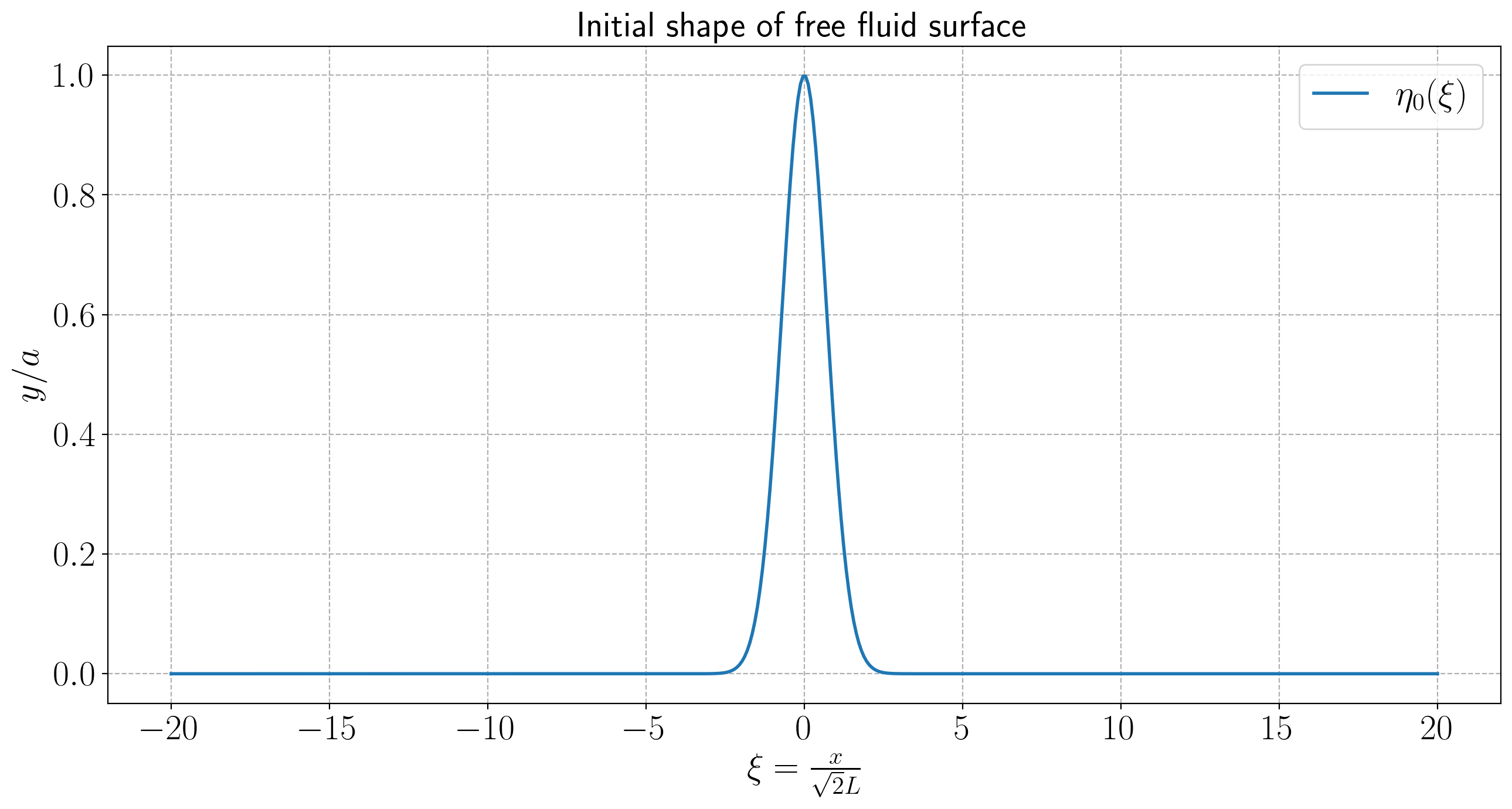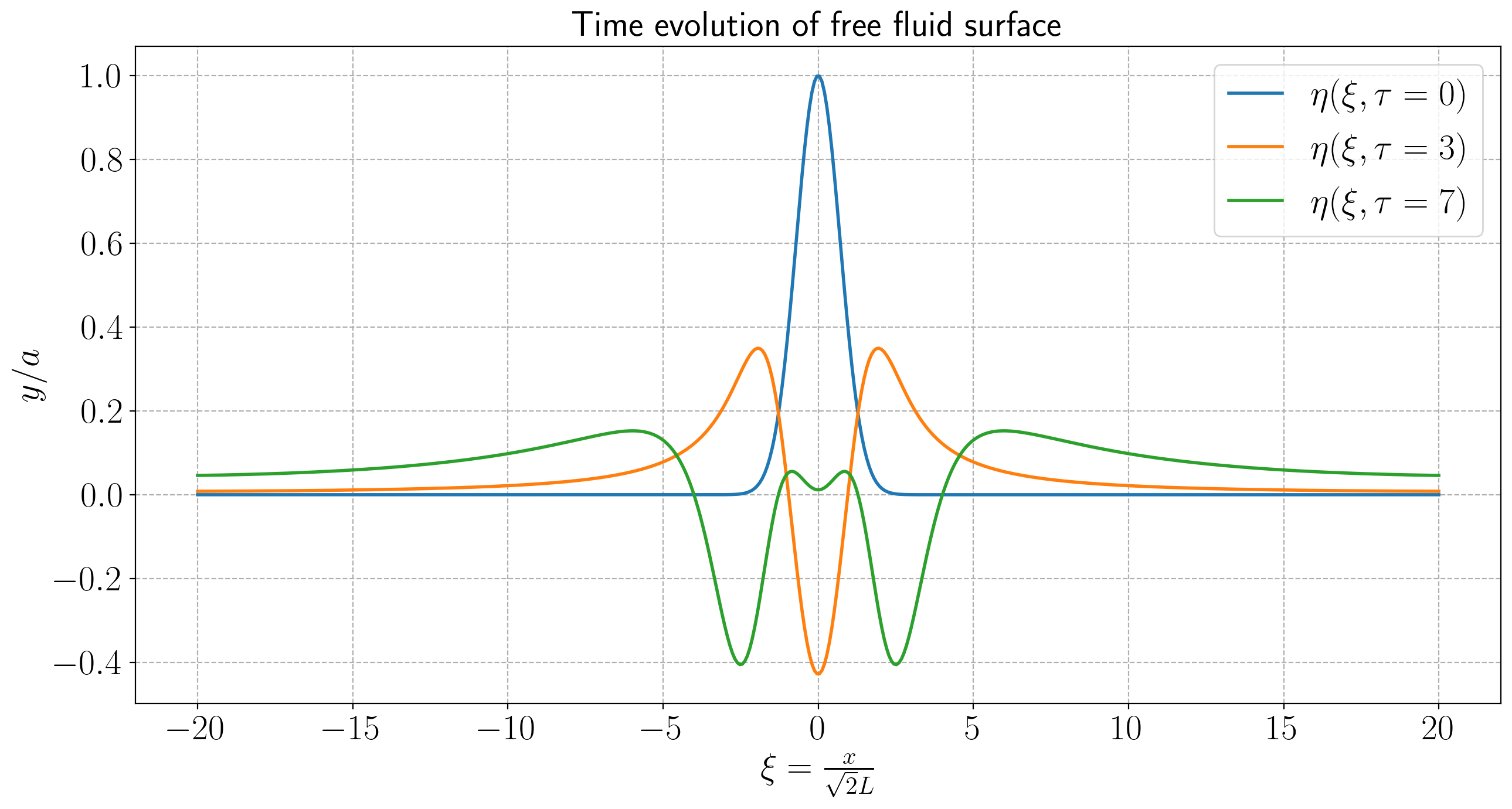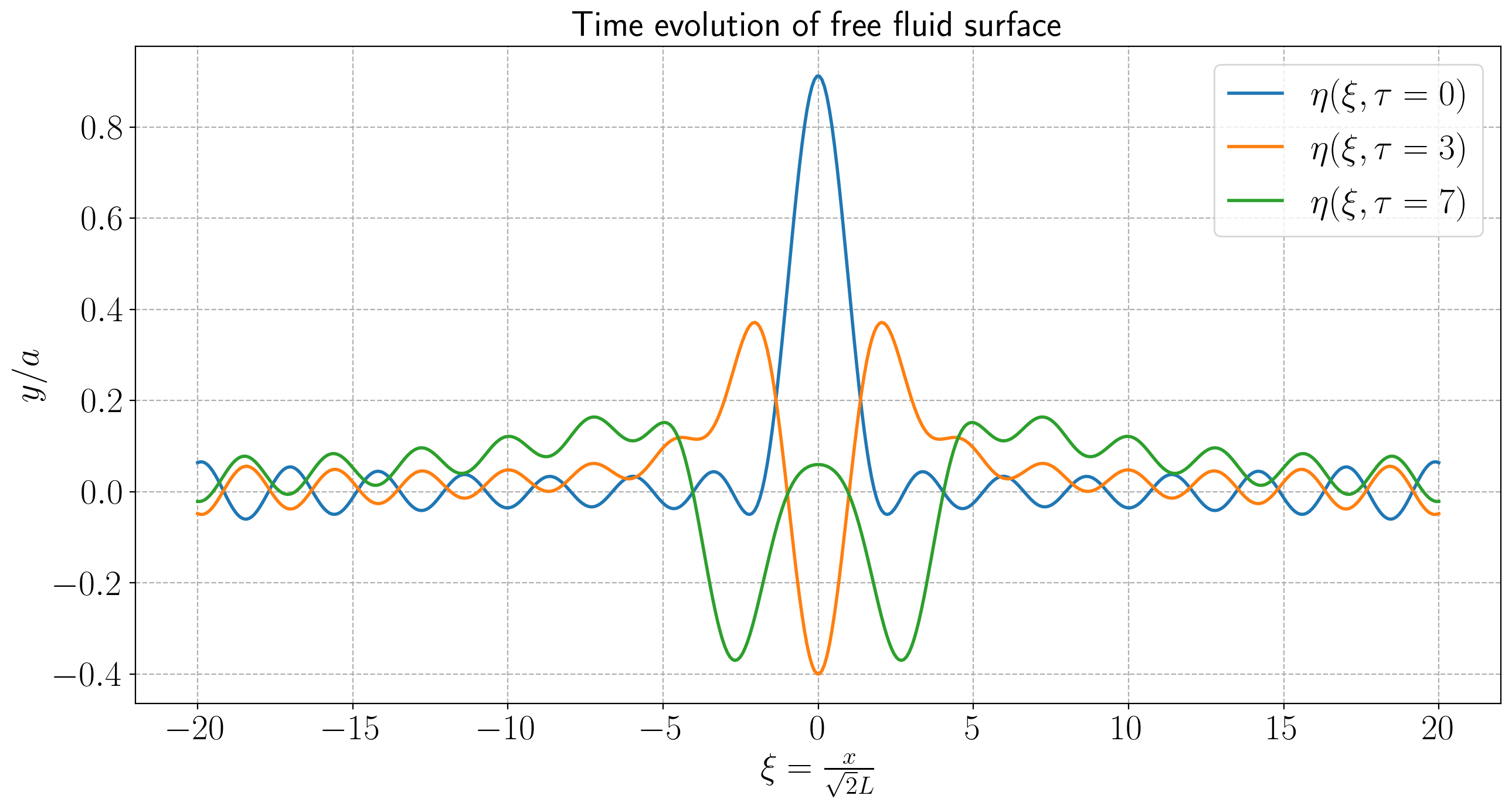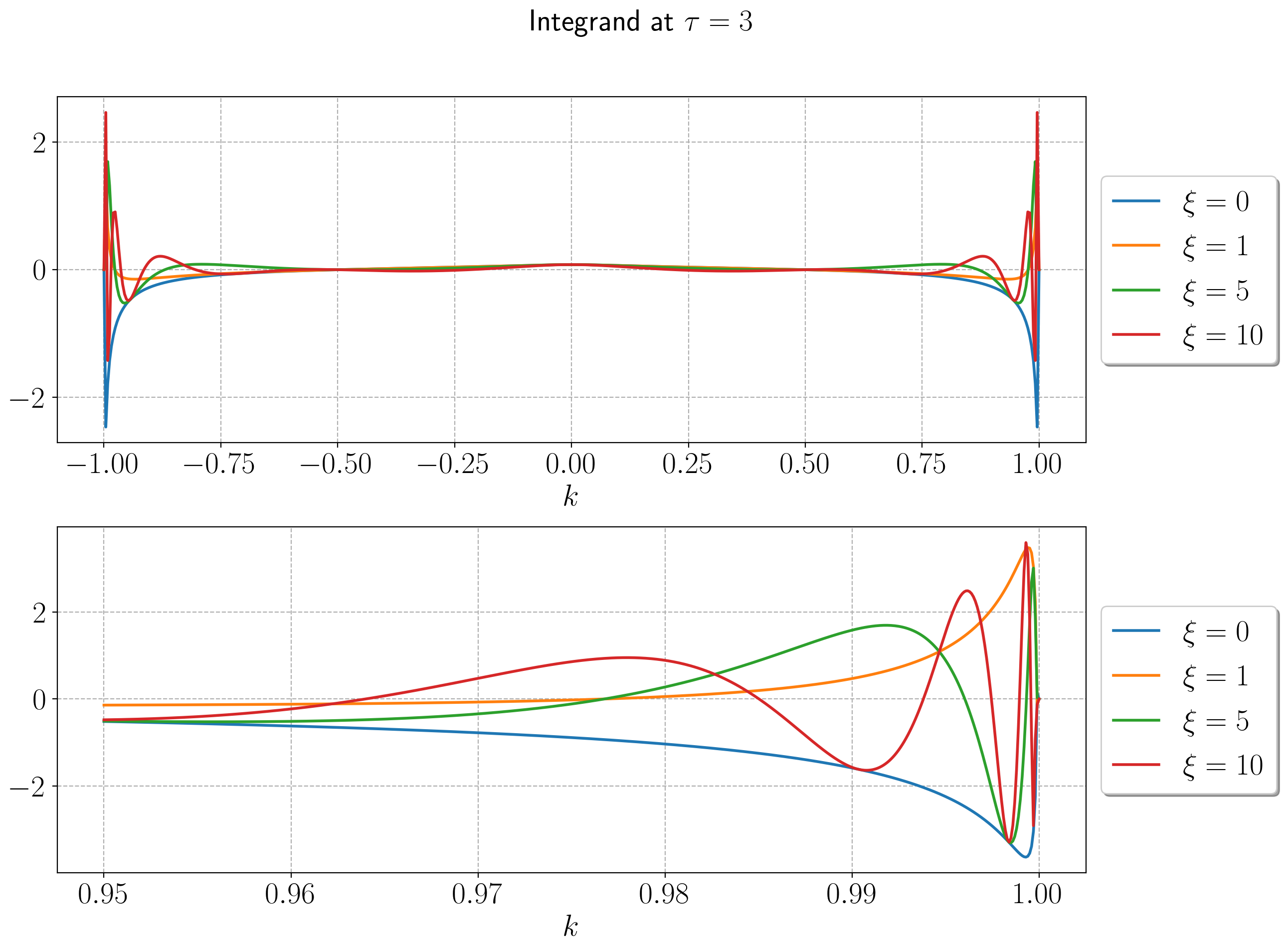# Linear wave propagation¶

### Examples - Fluid Mechanics¶

Last edited: October 13th, 2020

(This notebook is based on a problem given in the NTNU course TEP4105 - Fluid mechanics in the fall of 2019)

In this notebook, we will introduce some methods for analysing linear waves in infinite domains. In finite domains, separation of variables allows us to determine the natural modes of oscillation of the free fluid surface. Because of the linearity of the governing equations, any solution can be written as a superposition of such modes. In the infinite domain however, a general flow may be written as a superposition of harmonic waves. This creates an ideal environment for making use of the Fourier transform.

In particular, we will consider the problem of finding the time evolution of an initial perturbation $\eta_0(x)$ of the surface of an inviscid and incompressible fluid at constant depth.

## Properties of the Fourier transform¶

We present briefly some important properties of the Fourier transform which will be of use in this problem.

Let $f$ be a sufficiently well behaved function. We define the Fourier transform $\widehat{f}$ by

\begin{equation} \widehat{f} (k) = \frac{1}{\sqrt{2\pi}} \int_{-\infty}^{\infty} f(x) e^{-ikx} \, \text{d}x. \end{equation}

### Inverse Fourier transform¶

The original function $f$ is obtain by using the inverse transform defined by

\begin{equation} f(x) = \frac{1}{\sqrt{2\pi}} \int_{-\infty}^{\infty} \widehat{f}(k) e^{ikx} \, \text{d}x. \end{equation}

### Fourier transform of derivatives¶

As with the Laplace transform, the Fourier transform turns ordinary differential equations into algebraic equations, since derivatives with respect to $x$ are transformed into multiples of $ik$:

\begin{equation} \widehat{\frac{\text{d}^n f}{\text{d}x^n}} = \left(ik\right)^n \widehat{f}. \end{equation}

## Governing equations for Stokes waves¶

For small-amplitude, gravity-driven waves in an incompressible fluid we have the following governing equations for the velocity potential $\phi(x,y,t)$, and the free surface displacement $y = \eta(x,t)$ (see appendix for derivations).

\begin{align} \frac{\partial^2\phi}{\partial x^2} + \frac{\partial^2\phi}{\partial y^2} = 0& \quad \quad ; \quad \quad y < 0 \\ \frac{\partial\phi}{\partial y} = \frac{\partial \eta}{\partial t}, \quad \frac{\partial \phi}{\partial t} + g\eta = 0& \quad \quad ;\quad \quad y = 0 \\ \frac{\partial \phi}{\partial y} = 0& \quad \quad ; \quad \quad y = -h \end{align}

where $h$ is the mean water depth, and $g$ the acceleration of gravity. The first equation represents the continutiy equation for an incompressible fluid, namely $\mathbf{\nabla} \cdot \mathbf{v} = 0$, which translates to $\nabla^2\phi = 0$ for the velocity potential, in the case of irrotational flow. The two following equations are the kinematic and dynamic boundary conditions, and the last one is the impermeability at the bottom. We specify the initial conditions of the free fluid surface, and assume for simplicity that the surface is initially at rest

$$\eta(x,0) = \eta_0(x), \quad \frac{\partial\eta}{\partial t} = 0.$$

We take a Fourier transform of the above equations with respect to $x$. This yields the following

\begin{align} \frac{\partial^2 \widehat{\phi}}{\partial y^2} - k^2 \widehat{\phi} = 0& \quad \quad ; \quad \quad y < 0 \\ \frac{\partial \widehat{\phi}}{\partial y} = \frac{\partial \widehat{\eta}}{\partial t}, \quad \frac{\partial \widehat{\phi}}{\partial t} + g\widehat{\eta} = 0& \quad \quad ;\quad \quad y = 0 \\ \frac{\partial \widehat{\phi}}{\partial y} = 0& \quad \quad ; \quad \quad y = -h \\ \widehat{\eta} = \widehat{\eta_0}, \quad \frac{\partial \widehat{\eta}}{\partial t} = 0& \quad \quad ; \quad \quad t = 0 \end{align}

The solutions of the first equation is a linear combination of exponentials, which together with the impermeability condition in the third equation yields

\begin{equation} \widehat{\phi} = A(k,t) \cosh{ \left(k ( y + h)\right)}, \end{equation}

where the amplitude may depend on $k$ and $t$. From the second equation, the amplitude satisfies

\begin{equation}\label{eq:a1} Ak \sinh{kh} = \frac{\partial \widehat{\eta}}{\partial t} \end{equation}

and

\begin{equation}\label{eq:a2} \frac{\partial A}{\partial t}\cosh{kh} + g\widehat{\eta} = 0. \end{equation}

By differentiating equation \eqref{eq:a1} with respect to time, we get $\frac{\partial A}{\partial t} k \sinh{kh} = \frac{\partial^2\widehat{\eta}}{\partial t^2}$, which we can insert into equation \eqref{eq:a2} to get

$$\frac{\partial^2\widehat{\eta}}{\partial t^2} + gk \tanh{kh} \cdot \widehat{\eta} = 0.$$

This is the equation of simple harmonic motion. Upon applying the initial conditions, we obtain an expression for $\widehat{\eta}$

\begin{equation} \widehat{\eta} = \widehat{\eta_0} \cos{\omega t} \end{equation}

where $$\omega(k) = \sqrt{gk \tanh{kh}}$$ is the dispersion relation. The time evolution of the free fluid surface is obtained by applying the Fourier inversion theorem,

\begin{equation}\label{eq:eta} \eta(x,t) = \frac{1}{\sqrt{2\pi}} \int_{-\infty}^{\infty} \widehat{\eta_0}(k) \cos{\left(\omega(k) t\right)} e^{ikx} \, \text{d}k. \end{equation}

Unfortunately, finding an exact and explicit expression for $\eta(x,t)$ is hard, even for simple dispersion relations $\omega(k)$. In fact, solving this numerically is not entirely straightforward either, since it essentially involves solving two integrals over infinite domains. We need to solve both the integral in equation \eqref{eq:eta}, and the integral defining $\widehat{\eta_0}(k)$. To this end, we will present some approaches to obtain $\eta(x,t)$ numerically and analytically. We restrict our attention to a Gaussian initial perturbation $\eta_0(x) = a \exp{\left(- x^2 /2L^2 \right)}$.

## Gaussian perturbation¶

For the initial shape of the free fluid surface being $\eta_0(x) = a \exp\left(-x^2/2L^2\right)$ we get away with solving only one of the integrals, since $\widehat{\eta_0}$ can be calculated.

\begin{equation} \widehat{\eta_0}(k) = \frac{1}{\sqrt{2\pi}} \int_{-\infty}^{\infty} a \exp{\left(-\frac{x^2}{2L^2}\right)} \exp{(ikx)} \, \text{d} x = aL \exp{\left(-\frac{k^2L^2}{2}\right)}. \end{equation}

If we insert this into equation \eqref{eq:eta} we get

\begin{equation}\label{eq:eta2} \eta(x,t) = \int_{-\infty}^{\infty} \frac{\text{d}k}{\sqrt{2\pi}} aL\exp{\left(-\frac{k^2L^2}{2}\right)} \cos{\omega(k) t}\exp{(ikx)}. \end{equation}

When dealing with this expression, it is useful to introduce dimensionless quantities to represent $x$ and $t$. A natural time scale of this system is $\sqrt{L/g}$ and a useful length scale is $\sqrt{2}L$. This allows us to define the following dimensionless quantities

\begin{equation} \xi = \frac{x}{\sqrt{2}L} \quad ; \quad \tau = \frac{t}{\sqrt{L/g}} \end{equation}
In :
# Importing useful packages

import numpy as np
import matplotlib.pyplot as plt
import matplotlib as mpl
from matplotlib import rc
import matplotlib.gridspec as gridspec

eps = np.finfo(float).eps  # Machine decimal rounding

# Setting common plotting parameters
fontsize = 22
newparams = {'axes.titlesize': fontsize, 'axes.labelsize': fontsize,
'lines.linewidth': 2, 'lines.markersize': 7,
'figure.figsize': (13, 7), 'ytick.labelsize': fontsize,
'xtick.labelsize': fontsize, 'legend.fontsize': fontsize,
'legend.handlelength': 1.5, 'figure.titlesize': fontsize,
'figure.dpi':200, 'text.usetex': True, 'font.family': 'sans-serif'}
plt.rcParams.update(newparams)

In :
# Physical constants

g = 10    # Acceleration of gravity

global h  # Making h global so we can change it afterwards easily
h = 10    # Depth of water

a = 1     # Initial height of gaussian perturbation

global L  # Making L global so we can change it afterwards easily
L = 0.5   # Initial width of gaussian

t_0 = np.sqrt(L/g)  # Time scale
x_0 = np.sqrt(2)*L  # Length scale in x-direction

In :
def plot_waves(X,Y,title,labels):
"""
Function for plotting waves.

Args:
X (np.array)      : x-values for wave
Y (np.array)      : y-values for wave. Each row represents one wave.
title (string)    : title of plot
labels (np.array) : array of labels corresponding to each wave
"""

fig = plt.figure()
plt.title(title)

for i in range(Y.shape):
plt.plot(X,Y[i,:],label = labels[i])

plt.xlabel(r"$\xi = \frac{x}{\sqrt{2}L}$")
plt.ylabel(r"$y / a$")

plt.grid(linestyle="--")

plt.tight_layout()
plt.legend()

In :
def f(xi):
"""
Initial gaussian disturbance.

"""
return np.exp(-xi**2)

X = np.linspace(-20,20,500)
plot_waves(X,np.array([f(X)]),title = r"Initial shape of free fluid surface", labels = [r"$\eta_0(\xi)$"])## Changing the bounds on the integral¶

Since the infinite integration domain is difficult to deal with numerically, we might try to replace $\infty$ in the bounds by some large number, say $M$. After all, when we are dealing with a sharply localised initial wave, the integrand has to decay rather quickly, and the error made from introducing such restriction should therefore be small when the wave packet is sufficiently localised (ie. at short times after the perturbation occurred). In this context we have chosen to use $M = 50$ with $500$ panels on the trapezoid approximation. For more information on this algorithm, consult this this notebook.

In :
global M
M = 50

In :
# The decorator @np.vectorize vectorizes the function so that it can take as
# argument a nested sequence of objects or numpy arrays as inputs and returns a single numpy array
# for the documentation, see: https://numpy.org/doc/stable/reference/generated/numpy.vectorize.html

@np.vectorize
def comp_trap(f,a,b,N = 500):
"""
Simple implementation of the trapezoid rule.

Args:
f (function) : integrand
a (float)    : start point
b (float)    : end point
N (int)      : number of intervals, default = 500

Returns:
I (float)    : trapezoid integral approximation

"""
I = 0
delta_x = (b-a)/N
for i in range(N):
I += 0.5*delta_x*(f(a+delta_x*i)+f(a+delta_x*(i+1)))
return I

In :
def omega_grav(k):
"""
Function for gravity wave dispersion relation, omega(k)

"""
return np.sqrt(g*k*np.tanh(k*h))

In :
def eta_crude(xi,tau,N = 500,omega = omega_grav):
"""
Function for determining eta(xi,tau) using the crude
trapezoid approximation with the upper bounds changed from infinity to M

Args:
xi (float)       : xi-value(s)
tau (float)      : tau-value
N (int)          : number of intervals to use in trapezoid approx, default = 500
omega (function) : dispersion relation, default = omega_grav

Returns:
eta(xi,tau)
"""
prefac = L /(np.sqrt(2*np.pi))

def integrand(k):
return prefac * np.exp(-k**2*L**2/2)*np.cos(omega(k)*tau*t_0)*np.exp(1j*k*xi*x_0)

return comp_trap(integrand,-M,M,N = N)

In :
Y1 = eta_crude(X,0)
Y2 = eta_crude(X,3)
Y3 = eta_crude(X,7)

Y = np.array([np.real(Y1),np.real(Y2),np.real(Y3)])

plot_waves(X, Y,title = r"Time evolution of free fluid surface",
labels = [r"$\eta(\xi,\tau = 0)$",r"$\eta(\xi,\tau = 3)$",r"$\eta(\xi,\tau = 7)$"])To save some time, we include one method of doing the crude trapezoid approximation using more of the functionality offered by $\texttt{NumPy}$. To write the comp_trap-function using the $\texttt{Numpy}$ approach, one could do something similar to what is shown in the function comp_trap_numpy in the cell below. However, since we want to be able to call the function $\eta$ on an array of $\xi$-values, we have to rewrite the entire function, since the broadcasting features of $\texttt{NumPy}$ don't do what we intend in this context. The natural structure for solving this problem in $\texttt{NumPy}$ is meshgrids, see eg. this notebook for a detailed overview. The simple test below indeed confirmes that this method is faster, and we will therefore use this method when dealing with the composed trapezoid rule.

In :
def comp_trap_numpy(f, a, b, N=500):
"""This function is not used!
It is an example of how one could write comp_trap using NumPy.
However, simply swapping comp_trap with comp_trap_numpy in eta_crude
does not work, as NumPy broadcasting does not do what we intend it to in this context.
Thus, one has to rewrite eta_crude as well.
"""
x, dx = np.linspace(a, b, N, retstep=True)
I = dx * (np.sum(f(x)) - 0.5*f(x) - 0.5*f(x[-1]))
return I

In :
def eta_crude_numpy(xi,tau, N = 500, omega = omega_grav):
"""
Function for determining eta(xi,tau) using the crude
trapezoid approximation with the upper bounds changed from infinity to M,
using more of the functionality provided by NumPy.

Args:
xi (float)       : xi-value(s)
tau (float)      : tau-value
N (int)          : number of intervals to use in trapezoid approx, default = 500
omega (function) : dispersion relation, default = omega_grav

Returns:
eta(xi,tau)
"""
prefac = L /(np.sqrt(2*np.pi))

def integrand(k):
return prefac * np.exp(-k**2*L**2/2)*np.cos(omega(k)*tau*t_0)*np.exp(1j*k*xi*x_0)

k, dk = np.linspace(-M,M,N,retstep = True)

xixi, kk = np.meshgrid(xi,k)
integrand_mesh = integrand(kk)

I = dk * (np.sum(integrand_mesh, axis=0) - 0.5*integrand_mesh[0, :] - 0.5*integrand_mesh[-1, :])

return I

In :
# We use the timeit module to measure the execution times.
%timeit eta_crude(X, 0)
%timeit eta_crude_numpy(X, 0)

65.6 ms ± 3.1 ms per loop (mean ± std. dev. of 7 runs, 10 loops each)
14.1 ms ± 259 µs per loop (mean ± std. dev. of 7 runs, 100 loops each)


## Transforming the integral to a finite domain¶

Another approach to deal with the infinite domain is to introduce a change of variables, say $\zeta(k)$ such that $\zeta(\pm\infty)$ is finite. There are many choices for such a mapping, but lets try $\zeta (k) = \tanh(k)$ to begin with. Then $\text{d}\zeta = (1- \tanh^2k)\,\text{d}k = (1-\zeta^2)\,\text{d}k$ and $\zeta (k = \pm\infty) = \pm 1$. This turns the expression for the free fluid surface at a time $t$ into

\begin{equation*} \eta(x,t) = \int_{-1}^{1} \frac{\text{d}\zeta}{\sqrt{2\pi}} \frac{aL}{1-\zeta^2} \exp{\left(- \frac{\text{artanh}^2(\zeta)L^2}{2}\right)} \cos{\left(\omega(\text{artanh }(\zeta))t\right)} \exp{\left(i\text{artanh}(\zeta)x\right)}. \end{equation*}

When doing this integral numerically, it is obvious that we will have to avoid $\zeta = \pm 1$. We achieve this by changing our integration interval to $\zeta \in [-1 + 2 \epsilon, 1 - 2\epsilon]$, where $\epsilon$ is the machine epsilon.

In :
def eta_exact(xi,tau,N = 500, omega = omega_grav):
"""
Function for determining eta(xi,tau) using the 'exact' method of
mapping the coordinates to [-1,1], and then using trapezoid method to calculate integral

Args:
xi (float)       : xi-value(s)
tau (float)      : tau-value
N (int)          : number of intervals to use in trapezoid approx, default = 500
omega (function) : dispersion relation, default = omega_grav

Returns:
eta(xi,tau)
"""
def integrand(zeta):
return L/(np.sqrt(2*np.pi)) * 1/(1-zeta**2) * np.cos(omega(np.arctanh(zeta))*tau*t_0) * np.exp(- np.arctanh(zeta)**2 * L**2 /2 + 1j * xi * x_0 * np.arctanh(zeta))

# We add machine epsilon, eps, to avoid the endpoints xi = +-1.
k, dk = np.linspace(-1 + 2*eps,1-2*eps,N,retstep = True)

xixi, kk = np.meshgrid(xi,k)
integrand_mesh = integrand(kk)

I = dk * (np.sum(integrand_mesh, axis=0) - 0.5*integrand_mesh[0, :] - 0.5*integrand_mesh[-1, :])

return I

In :
Y1 = eta_exact(X,0)
Y2 = eta_exact(X,3)
Y3 = eta_exact(X,7)

Ys = np.array([np.real(Y1),np.real(Y2),np.real(Y3)])

plot_waves(X, Ys,title = r"Time evolution of free fluid surface",
labels = [r"$\eta(\xi,\tau = 0)$",r"$\eta(\xi,\tau = 3)$",r"$\eta(\xi,\tau = 7)$"])When using exactly the same, naive trapezoid rule on this integral, we clearly see that this produces results that are very unsatisfactory. For small $\xi$, the results are ok, but get worse as $\xi$ increases. By plotting the integrand at (say) $\tau = 3$ for some different $\xi$, we can get an idea what this is caused by.

In :
def integrand(zeta,xi,tau):
return L/(2*np.pi) * 1/(1-zeta**2) * np.cos(omega_grav(np.arctanh(zeta))*tau*t_0) * np.exp(- np.arctanh(zeta)**2 * L**2 /2 + 1j * xi * x_0 * np.arctanh(zeta))

Z1 = np.linspace(0.95,1 - eps,500)    # array of zeta values between 0.95 and 1-eps
Z2 = np.linspace(-1+ eps,1 - eps,500) # array of zeta values between -1 + eps and 1 + eps

# Integrand evaluated at different \xi values on the short interval

I1_short = integrand(Z1,0,3)
I2_short = integrand(Z1,1,3)
I3_short = integrand(Z1,5,3)
I4_short = integrand(Z1,10,3)

# Integrand evaluated at different \xi values on the large interval

I1 = integrand(Z2,0,3)
I2 = integrand(Z2,1,3)
I3 = integrand(Z2,5,3)
I4 = integrand(Z2,10,3)

fig = plt.figure(figsize=(13,10))

fig.suptitle(r"Integrand at $\tau = 3$")

gs = gridspec.GridSpec(2,1) # Making a 2 x 1 grid

ax = plt.subplot(gs[0,:])   # First axis is the first row

ax.plot(Z2,np.real(I1),label = r"$\xi = 0$")
ax.plot(Z2,np.real(I2),label = r"$\xi = 1$")
ax.plot(Z2,np.real(I3),label = r"$\xi = 5$")
ax.plot(Z2,np.real(I4),label = r"$\xi = 10$")

plt.xlabel(r"$k$")
plt.grid(linestyle="--")

# Placing legend outside plot
ax.legend(loc='center left', bbox_to_anchor=(1, 0.5),

ax = plt.subplot(gs[1,:])    # Second axis is the second row

ax.plot(Z1,np.real(I1_short),label = r"$\xi = 0$")
ax.plot(Z1,np.real(I2_short),label = r"$\xi = 1$")
ax.plot(Z1,np.real(I3_short),label = r"$\xi = 5$")
ax.plot(Z1,np.real(I4_short),label = r"$\xi = 10$")

# Placing legend outside plot
ax.legend(loc='center left', bbox_to_anchor=(1, 0.5),

plt.xlabel(r"$k$")
plt.grid(linestyle="--")

plt.tight_layout(rect=[0, 0.03, 1, 0.95]) # tight_layout adjusted to work with suptitleThe integrand varies very rapidly near $\pm 1$ for increasing $x$, and it therefore becomes hard to accurately integrate it with equidistant integration abscissae. We can try to resolve this problem by not using uniformly distributed integration points, but rather let more of them be situated near $\pm 1$. Alternatively, we can choose our coordinate mapping more cleverly, which allows us to convert the problem into one of calculating a Fourier series. This is what we will consider in the following.

### Fourier - Chebyshev quadrature¶

Another way to map the coordinates to a finite interval is to consider a transformation of the form $k = \chi(\theta) = J \cot{\theta}$, effectively transforming our integration interval to $[0,\pi]$. This can be seen from the fact that $\chi'(\theta) = -J \frac{1}{\sin^2(\theta)}$, and that $\chi(\theta = \pi) = -\infty$ and $\chi(\theta = 0) = \infty$. It follows that \begin{equation*} \eta(\xi,\tau) = \int_{-\infty}^{\infty} f (k;\xi,\tau)\, \text{d}k = J \int_{0}^{\pi} \text{d}\theta \frac{f(J \cot{\theta};\xi,\tau)}{\sin^2{\theta}}, \end{equation*}

where $J$ is a user-defined constant. One advantage associated to mapping it onto $[0,\pi]$ is that it is straightforward to compute the integral of sines and cosines on this interval. Therefore, we try to expand the integrand in its Fourier series and then integrate it. The idea is the following (see eg. ):

We want to calculate the integral

\begin{equation}\label{eq:int} I = \int_{0}^{\pi} f[\chi(\theta)] \chi'(\theta) \, \text{d}\theta, \end{equation}

where $\chi$ is the function that maps our interval $[-\infty,\infty]$ to $[0,\pi]$. In our case, we choose $\chi(\theta) = J \cot{\theta}$. Notice that since this function actually maps the upper bound ($+ \infty$) to $0$ and the lower bound to $\pi$ but in addition carries a minus sign in the derivative, the above notation $\chi'(\theta)$ is somewhat abusive since it disregards the minus sign in the derivative.

Suppose we approximate the integrand $f(\chi(\theta))$ by its truncated Fourier series expansion through trigonometric interpolation, that is

\begin{equation}\label{eq:trig_interpolate} q(\theta) = a_0 + \sum_{n=1}^N a_n \cos{n \theta} + \sum_{n=1}^{N} b_n \sin{n \theta}, \end{equation}

where $N$ is some appropriately chosen cut-off point.

Now suppose we combine the trigonometric basis functions into new cardinal functions $C_j$ for each interpolation point $t_j$ with the property that

\begin{equation}\label{eq:cardinal_funcs} C_j(t_i) = \begin{cases} 1, i = j\\ 0, i \neq j \end{cases}. \end{equation}

The explicit form of these cardinal functions can be found in the appendix. When inserted into equation \eqref{eq:trig_interpolate}, this yields

$$q(\theta) = \sum_{j=0}^{N-1} q(t_j) C_j(\theta).$$

The last step is to integrate $q(\theta)$ to approximate the integral we are interested in solving in \eqref{eq:int},

$$I \approx \sum_{j=0}^{N-1} w_j f (\chi(t_j)),$$

where the weights $w_j$ are defined as $$w_j = \chi'(t_j) \int_{0}^{\pi} C_j(\theta)\, \text{d}\theta.$$

When using the mapping $k \mapsto J \cot{\theta}$, one can calculate the weights, $w_j$ , and the approximation for $N$ interpolation points reads

\begin{equation*} \eta(\xi,\tau) \approx \frac{J\pi}{N-1} \sum_{n = 1}^{N-2} \frac{f(J \cot{(\pi n/(N-1))};\xi,\tau)}{\sin^2{(n\pi/(N-1))}} + \frac{J\pi}{2N-2} \left( \frac{f(J \cot{(0)};\xi,\tau)}{\sin^2{(0)}} + \frac{f(J \cot{(\pi)};\xi,\tau)}{\sin^2{(\pi)}} \right), \end{equation*}

where we assume the two last terms in the parantheses are finite. The derivation of these formulae can be found in the article referred to in , and some details are presented in the appendix. To avoid division by $0$-issues, we evaluate $\sin$ and $\cot$ at $\epsilon$ and $\pi - \epsilon$ instead of $0$ and $\pi$, where $\epsilon$ is the machine limit for decimal rounding.

In :
def eta_exact_good(xi,tau,J = 10 ,N = 500,omega = omega_grav):
"""
Function for determining eta(xi,tau) using the Fourier chebyshev method,
with coordinate mapping k = J cot (theta) in the integral.

Args:
xi (float)       : xi-value(s)
tau (float)      : tau-value
n (int)          : number of intervals to use in trapezoid approx, default = 500
omega (function) : dispersion relation, default = omega_grav

Returns:
eta(xi,tau)
"""
prefac = L /(np.sqrt(2*np.pi))

def integrand(k):
return prefac * np.exp(-k**2*L**2/2)*np.cos(omega(k)*tau*t_0)*np.exp(1j*k*xi*x_0)

S1 = sum(J*np.pi/(N-1) * integrand(J*1/np.tan(n*np.pi/(N-1)))*1/(np.sin(n*np.pi/(N-1))**2) for n in range(1,N-1))
S2 = J*np.pi/(2*N-2)*integrand(J*1/np.tan(eps))*1/((np.sin(eps))**2)
S3 = J*np.pi/(2*N-2)*integrand(J*1/np.tan(np.pi - eps))*1/((np.sin(np.pi - eps))**2)

return S1 + S2 + S3


In :
Y1 = eta_exact_good(X,0)
Y2 = eta_exact_good(X,3)
Y3 = eta_exact_good(X,7)

Ys = np.array([np.real(Y1),np.real(Y2),np.real(Y3)])

plot_waves(X,Ys,title = r"Time evolution of free fluid surface",
labels = [r"$\eta(\xi,\tau = 0)$",r"$\eta(\xi,\tau = 3)$",r"$\eta(\xi,\tau = 7)$"])